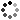# Mathematical Artwork in Python Ebook and Code

On Sale
\$40.00
\$40.00
Preview
Learn how to write Python code to create mathematically-based artwork and fractals like the ones on codeismycanvas. This e-book, provided in HTML and PDF formats, provides simple Python code, relying only on common libraries such as Numpy, Pillow, and Matplotlib, so the reader can fully understand the math behind the images. The book intends to bridge the gap between math, code, and design. The book assumes a basic knowledge of Python and some high-school level math.

Included with the book are a number of extras:
• Jupyter notebook with interactive controls for exploring Mandelbrot and Julia Sets by escape time coloring.
• Jupyter notebook with interactive controls for exploring Orbit Trap fractals.
• Interactive Jupyter notebook for exploring Lissajous curves, harmonograph equation drawings, and spirograph equation drawings.
• Interactive notebook for creating and exploring strange attractor plots.
• Code for generating FFT-based sketches (in the style of Wolfram), and their parametric equations, by point-and-click.
• Jupyter notebook for interactively creating color palettes
• A list of 400 points in the complex plane, listed to 17 digits of precision, that iterate the Mandelbrot equation at least 1 million and up to 5 million times before escaping. Save your CPU when making high-resolution Buddhabrot fractals.

Second Edition: The book was updated in November 2023 to ensure the code all runs properly and efficiently under the latest versions of Python. The Interactive Notebooks were also updated, including addition of an Orbit Trap Fractal interactive notebook.

License: Any original work you create using the code and ideas in the book are your own and may be sold or distributed as you like. The book, code, and all other contents of this product may not be resold or distributed.
You will get the following files:
• PDF (21MB)
• ZIP (40MB)
• ZIP (2MB)